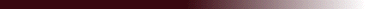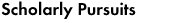This group of papers deals with the analytical uses of continuous wavelet transforms. As it turns out, Hermitian wavelets (the `Mexican hat' family) are fundamentally related to the diffusion operator.

"Formal improvements in the solution of the wavelet-transformed Poisson and diffusion equations.

to appear, J. Math. Phys. (1998)

"A Hamiltonian Formulation for the Diffusion Equation"

Physical Review E 55, 1590-1599 (1997)

"Wavelet Transforms of some Equations of Fluid Mechanics"

Acta Mechanica 104, 1-25, 1994.

"Wavelet Transforms of the Navier-Stokes Equations and the Generalized Dimensions of Turbulence"

Appl. Sci. Res. 51, 109-113, 1993.

"Energy Dissipation in the Wavelet-Transformed Navier-Stokes Equations"

Phys. Fluids A5, 1512-1513, 1993.

"On the effect of boundary conditions on the multifractal statistics of incompressible turbulence"

IMA Conference on Multiscale Stochastic Processes Analyzed using multifractals and wavelets, 1993.

Jacques Lewalle, jlewalle@syr.edu